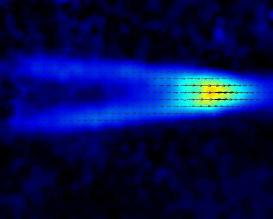## Vertical length scale selection for pancake vortices in strongly stratified viscous fluidsVertical length scale selection for pancake vortices in strongly stratified viscous fluids
R. Godoy-Diana; J. M. Chomaz & P. Billant.
Journal of Fluid Mechanics, 504 : 229-238 (2004).

Abstract: The evolution of pancake dipoles of different aspect ratio is studied in a stratified tank experiment. Two cases are reported here for values of the dipole initial aspect ratio $\alpha_0 = L_v/L_h$ (where $L_v$ and $L_h$ are vertical and horizontal length scales, respectively) of $\alpha_0 = 0.4$ (case I) and $\alpha_0 = 1.2$ (case II). Continue reading “Vertical length scale selection for pancake vortices in strongly stratified viscous fluids”